Our World Statistics Day conversations have been a great reminder of how much statistics can inform our lives. Do you have an example of how statistics has made a difference in your life? Share your story with the Community!
Choose Language Hide Translation Bar
Highlighted

## Plot 2D Trace

Is it possible to generate a 2D trace in JMP? I.e., plot a list of x/y coordinates, connecting consecutive points with a line, as shown in the attached PNG?

6 REPLIES 6
Highlighted

## Re: Plot 2D Trace

Something like:

NamesDefaultToHere(1);

n= 10;

x = J(n, 1, RandomInteger(0, 100));

y = J(n, 1, RandomInteger(0, 100));

NewWindow("XY Trace", GraphBox(PenColor("Red"), Line(x,y)));

Look in the JSL Guide or the Scripting Index for more details on the options for 'GraphBox()'. To get values from a column in a table into a matrix send the column a 'GetAsMatrix()' message.

Highlighted

## Re: Plot 2D Trace

Thanks lots!

Highlightedlouv
Staff (Retired)

## Re: Plot 2D Trace

I used the Bubble Plot platform...

//Create a table with the drawing points, repeat that origin (start and finish)

drawDT = New Table( "Draw Plot",

//Set the values for the coordinates to the table

New Column( "X", Numeric, "Continuous", Format( "Best", 12 ), Set Values( [0, 0.3, 0.7, 1, 0.4, 0] ) ),

New Column( "Y", Numeric, "Continuous", Format( "Best", 12 ), Set Values( [0, 0.9, 1, 0.1, 0.3, 0] ) ),

New Column( "Draw Order", Numeric, "Continuous", Format( "Best", 12 ), Set Values( [1, 2, 3, 4, 5, 6] ) )

);

//Create a new window with bubble plot

New Window( "Draw Plot",

//On close of the window, the data table is deleted

<<on close( Close( drawDT ) ),

Bubble Plot( X( :X ), Y( :Y ), Time( :Draw Order ), Time Index( 6 ), Trail Bubbles( "All" ), Trail Lines( "All" ), )

);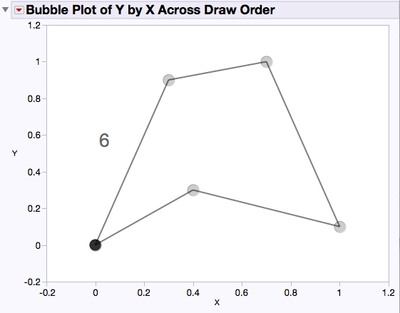Highlighted

Thanks lots!

Highlighted

## Re: Plot 2D Trace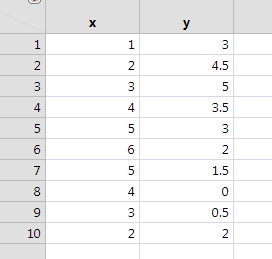Open graph builder and drag the variables to the axes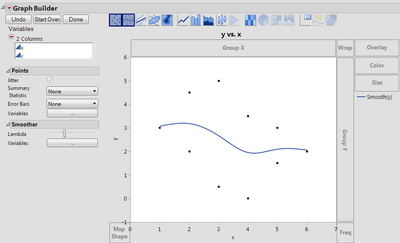turn off the points and smoother and turn on the line and select row order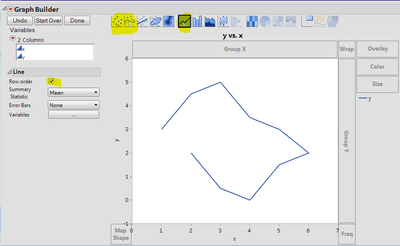click done and save the script (red triangle) if you want to run it again without all the drag-and-drop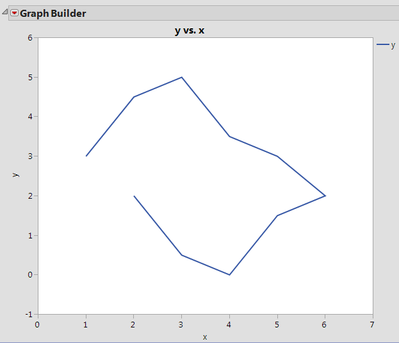`Graph Builder(  Show Control Panel( 0 ),  Variables( X( :x ), Y( :y ) ),  Elements(  Line( X, Y, Legend( 3 ), Row order( 1 ), Summary Statistic( "Mean" ) )  ))`

repeat the first point to close the loop if desired.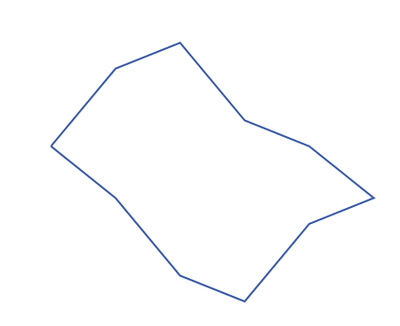Craige
Highlighted

## Re: Plot 2D Trace

Thanks lots!

Article Labels

There are no labels assigned to this post.